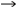# Using an H-Bridge Transformer Driver to Charge a Supercapacitor

### 要約

With its small size and large load (10W) capability, the MAX13256 H-bridge transformer driver is an attractive solution for charging supercapacitors (supercaps). However, a large capacitance on the output of the circuit can force the driver into fault mode at startup, due to the high initial charge current. This application note presents a solution that allows users to charge a large capacitance without going into fault.

Historically, supercapacitors (supercaps) have seen limited use in electronic circuits. Due to their large size and mediocre energy capacity, supercaps have primarily been used in low-power applications such as memory-power backup. In the last few years, however, significant advances have been made to improve the energy and power capability of supercaps, making them an increasingly attractive solution for a wider range of battery-based applications, including handheld devices, wireless products, and actuators.
With its small size and large load (10W) capability, the MAX13256 H-bridge driver is an attractive solution for charging supercaps while simultaneously driving a system load¹. However, a large capacitance placed directly on the output of the MAX13256 circuit can force the driver into fault mode at startup, due to the high charge current required when the capacitor is completely discharged. While a wide variety of acceptable current limiter circuits are available, many of these solutions waste power and may involve a significant voltage drop on the output. So, is there a way to make the circuit work without a significant loss of power?

### A Small and Simple Solution!

The addition of a small diode and resistor in parallel between the positive lead of the supercap and the output of the transformer circuit limits the current used to charge the supercap during normal operation and allows for easy discharge when energy is required (Figure 1). The diode is reversed-biased while the supercap is charging, and the charge current is limited by the parallel resistor, RLIMIT. The diode conducts when the supercap is discharging, resulting in a small voltage drop from the capacitor to the load. The diode does not otherwise limit the flow of charge out of the supercap.Figure 1. Diode-resistor current-limit circuit for charging a supercap with the MAX13256 H-bridge transformer driver.
Some care must be taken when calculating the value of RLIMIT in systems where the required system current (ISYSTEM) must be maintained while charging the supercap, CSUP. Ensure that the current to charge the supercap and the required system current do not exceed the current limit set by the MAX13256. See the MAX13256 data sheet for more information on setting the current limit for the device.
First, calculate the amount of current available to charge the supercap, ISUP_CHG, using the following equation:
ISUP_CHG = ILIMIT - ISYSTEM
where ILIMIT is the current limit set by the MAX13256.
RLIMIT limits the charge current into the supercap when it is completely discharged. Calculate RLIMIT using the following equation:
RLIMIT = VISO_MAX/ISUP_CHG
where VISO_MAX is the maximum voltage expected on the output of the transformer circuit.

### A Simple Example

Given the circuit in Figure 2, suppose that the maximum system load required is 700mA.Figure 2. Sample MAX13256 supercap charging circuit.
With the 2:1 drop on the transformer and a 1kΩ resistor on the ITH pin of the MAX13256, we can pull at least 1A from the secondary side of the circuit before the IC goes into fault mode. Using the equation above, we calculate:
ISUP_CHG = 1A - 700mA = 300mA
To limit the current into the supercap, RLIMIT, is:
RLIMIT = 5.5V/0.3A = 18.3Ω.
The nearest standard larger value would be 18.7Ω, thus RLOAD = 18.7Ω.
Finally, we can calculate the longest time needed² to charge the supercap.
Time to charge = 5Τ = 5 × RLIMIT × CSUP = 5 × 18.7Ω × 500mF = 47 seconds
So, it will take less than 1 minute to charge the supercap while driving the system load.

#### Notes:

1. While it should go without saying, it won't: the MAX13256 H-bridge driver can be used to charge "normal" capacitors as well as supercaps. 2. The calculation shown here is a general guideline for the time required to completely charge a capacitor. A more complex, but more precise approach would be to calculate the time required to charge using the standard:
iC = C × dV/dtdt = C × dV/iCt = (C/iC) × ∫dV
However, this assumes a true constant current source.